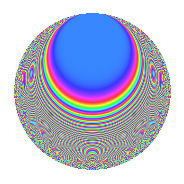# Properties

 Label 402.2.hLevel 402 Weight 2 Character orbit h Rep. character $$\chi_{402}(239,\cdot)$$ Character field $$\Q(\zeta_{6})$$ Dimension 44 Newform subspaces 2 Sturm bound 136 Trace bound 2

# Related objects

## Defining parameters

 Level: $$N$$ = $$402 = 2 \cdot 3 \cdot 67$$ Weight: $$k$$ = $$2$$ Character orbit: $$[\chi]$$ = 402.h (of order $$6$$ and degree $$2$$) Character conductor: $$\operatorname{cond}(\chi)$$ = $$201$$ Character field: $$\Q(\zeta_{6})$$ Newform subspaces: $$2$$ Sturm bound: $$136$$ Trace bound: $$2$$ Distinguishing $$T_p$$: $$5$$

## Dimensions

The following table gives the dimensions of various subspaces of $$M_{2}(402, [\chi])$$.

Total New Old
Modular forms 144 44 100
Cusp forms 128 44 84
Eisenstein series 16 0 16

## Trace form

 $$44q - 22q^{4} - q^{6} - 12q^{7} + 2q^{9} + O(q^{10})$$ $$44q - 22q^{4} - q^{6} - 12q^{7} + 2q^{9} - 3q^{12} + 12q^{13} + 8q^{15} - 22q^{16} - 3q^{18} - 6q^{19} - 6q^{21} - 24q^{22} + 2q^{24} + 20q^{25} + 12q^{28} - 6q^{30} + 48q^{31} - 6q^{33} + 6q^{34} - q^{36} - 20q^{37} - 12q^{39} - 60q^{46} + 3q^{48} + 54q^{49} - 3q^{51} - 28q^{54} - 20q^{55} - 3q^{57} - 4q^{60} - 12q^{61} - 42q^{63} + 44q^{64} - 40q^{67} + 30q^{69} - 18q^{73} + 12q^{76} + 48q^{78} - 24q^{79} + 82q^{81} - 56q^{82} - 6q^{84} + 60q^{85} + 18q^{87} + 12q^{88} + 26q^{90} - 8q^{91} - 18q^{93} - q^{96} + 138q^{97} - 78q^{99} + O(q^{100})$$

## Decomposition of $$S_{2}^{\mathrm{new}}(402, [\chi])$$ into newform subspaces

Label Dim. $$A$$ Field CM Traces $q$-expansion
$$a_2$$ $$a_3$$ $$a_5$$ $$a_7$$
402.2.h.a $$22$$ $$3.210$$ None $$-11$$ $$1$$ $$0$$ $$-6$$
402.2.h.b $$22$$ $$3.210$$ None $$11$$ $$-1$$ $$0$$ $$-6$$

## Decomposition of $$S_{2}^{\mathrm{old}}(402, [\chi])$$ into lower level spaces

$$S_{2}^{\mathrm{old}}(402, [\chi]) \cong$$ $$S_{2}^{\mathrm{new}}(201, [\chi])$$$$^{\oplus 2}$$